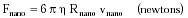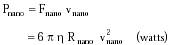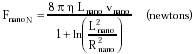Nanomedicine, Volume I: Basic Capabilities

Robert A. Freitas Jr., Nanomedicine, Volume I: Basic Capabilities, Landes Bioscience, Georgetown, TX, 1999

9.4.2.4 Force and Power Requirements

Consider a spherical nanorobot of radius Rnano that is falling at uniform velocity vnano through an incompressible (Newtonian) fluid of viscosity h. If the Reynolds number NR << 1 (Eqn. 9.65), then Stokes1373 found that the total force of resistance imparted on the sphere by the fluid is given by:{Eqn. 9.73}

also known as Stokes law. This result applies only in the case of a single sphere in an infinite expanse of homogeneous viscous fluid, and neglects all inertial terms. If the fluid container is finite in size, or if there are other spheres in the neighborhood, or if inertial forces cannot be entirely ignored because NR >~ 1 (e.g., Rnano = 1 micron, vnano > 1 m/sec in water), then the equation must be corrected.1374 Dynamic effects due to sphere oscillation, sudden release from rest, or variable speed also require corrections,1374 and there are other corrections for neighboring spheres.1375 Most of these corrections are of order near-unity for micron-scale objects.

Eqn. 9.73 provides a useful approximation of the motive force required to drive a spherical nanorobot through blood plasma. Assuming a perfectly efficient drive mechanism, the power requirement337 is at least:{Eqn. 9.74}

For example, a force of Fnano = 200 pN and a power of at least Pnano = 2 pW are required to drive a Rnano = 1 micron spherical nanorobot at vnano = 1 cm/sec through blood plasma at 310 K with h = 1.1 x 10-3 kg/msec. These formulas may also be used to estimate nanodevice velocity, given Fnano or Pnano.

Of course, drive mechanisms are not perfectly efficient. Propulsion efficiency is often poor for objects that swim at low Reynolds numbers (e.g., typically NR ~ 10-3 for flagellates).3578 A sphere driven by a helical propeller (e.g., flagellar propulsion; Section 9.4.2.5.2) of arbitrary length may have a propulsion efficiency as low as e% ~ 0.01(1%).389 Propulsive efficiency for organisms with spherical heads 10-40 times larger than their flagellar radius (optimum shape), using helical flagellar beats, ranges from e% = 0.10-0.28 (10%-28%),1377 requiring a propulsive input power of ~Pnano/e% ~ 7-20 pW in the previous example. Other drive mechanisms may prove more efficient.

Force requirements for nonspherical nanorobots are similar in magnitude. For example, consider a cylinder of radius Rnano and length Lnano translating uniformly in a direction normal to its axis. Lighthill1367,1376 shows that the force (applied at the midpoint) required to move this object at velocity vnano is given by:{Eqn. 9.75}

for Lnano >> Rnano.363 (If the perpendicular force is applied at a distance c from one end of the cylinder, then the Lnano2 term in Eqn. 9.75 is replaced by 4 c (Lnano - c) when c >> Rnano.) Eqn. 9.75 does not hold for a perpendicular force applied near the ends of the cylinder. Also, a constant Fnano actually produces a slowly varying velocity field along the cylinder length; for a midpoint-applied force, vnano is at maximum at the midpoint, but the percentage variation over most of the cylinder is not large.363 Experiments show that needle-shaped bodies fall in viscous media about half as fast sideways as they do end-on;1378 that is, the force on a long cylinder translating parallel to its axis is FnanoP ~ (1/2) FnanoN. The power requirement is then calculated as in Eqn. 9.74.

Interestingly, there seems to be a sharp minimum size limit of ~0.6 microns for free-swimming foraging microbes, below which size locomotion has no apparent benefit.3581 This theoretical conclusion is supported by the observation that the smallest 97 genera of motile bacteria have a mean length of 0.8 microns, whereas 18 of 94 nonmotile genera are smaller.3581

Last updated on 21 February 2003